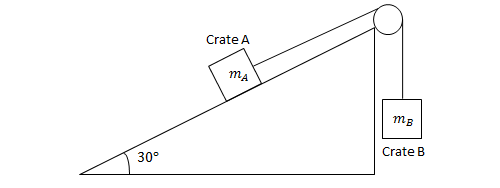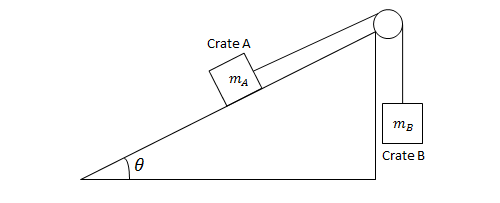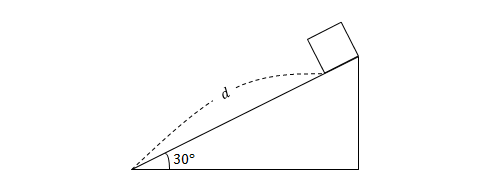Classical Mechanics

# Motion along Inclined PlanesIn the figure above, the inclined plane makes a $30^\circ$ angle with the horizontal. The masses of crates $A$ and $B$ are $m_A=80\text{ kg}$ and $m_B=x\text{ kg},$ respectively. The coefficient of kinetic friction at the surface of the inclined plane is $\mu=\frac{\sqrt{3}}{10},$ and the pulley is frictionless. If crate $A$ is sliding up the ramp at a constant speed, what is the value of $x?$

A block slides down an inclined plane that makes a $45^\circ$ angle with the floor. If the coefficient of kinetic friction is $\mu=\frac{1}{19},$ what is the acceleration of the block?

The gravitational acceleration is $g=10\text{ m/s}^2.$

A coin slides down a ramp angled at $30^\circ$ with respect to the horizontal. If the coin starts from rest, what is its speed in m/s after sliding $1~\mbox{m}$?

Details and assumptions

• The ramp is frictionless.
• The acceleration of gravity is $-9.8~\mbox{m/s}^2$.In the figure above, the inclined plane makes an angle $\theta$(in radians) with the horizontal. The masses of crates $A$ and $B$ are $m_A=17\text{ kg}$ and $m_B=6\text{ kg},$ respectively. If the ramp is perfectly frictionless, and crate $A$ slides down the ramp at a constant speed, what is the value of $\sin\theta?$A block slides down a frictionless, inclined plane that makes a $30^\circ$ angle with the floor. If the block is initially at rest, and the length of the inclined plane is $d=15\text{ m},$ how many seconds does it take for the block to reach the end of the plane?

The gravitational acceleration is $g=10\text{ m/s}^2.$

×